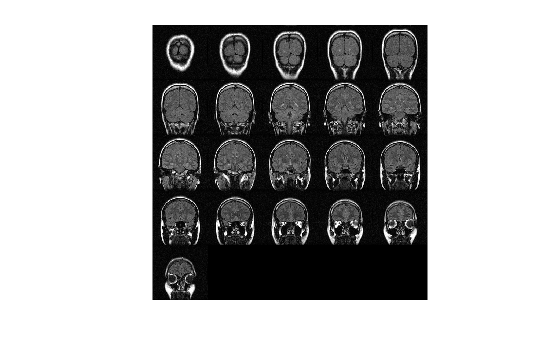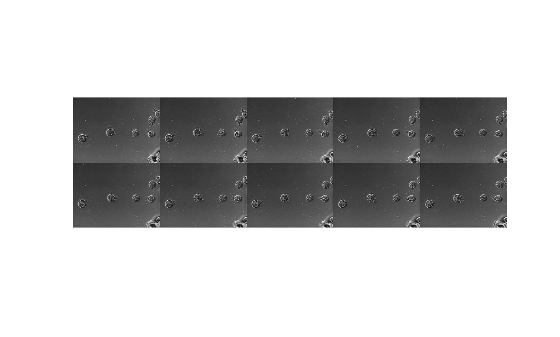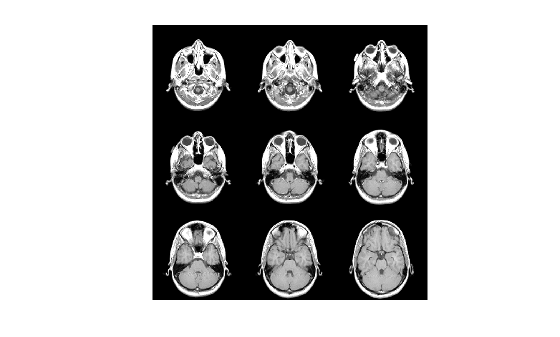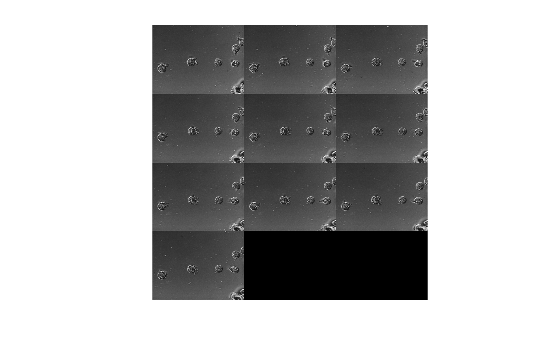# montage

Display multiple image frames as rectangular montage

## Syntax

``montage(I)``
``montage(imagelist)``
``montage(filenames)``
``montage(imds)``
``montage(___,map)``
``montage(___,Name,Value)``
``img = montage(___)``

## Description

example

````montage(I)` displays all frames of a multiframe image array `I`. By default, `montage` arranges the images so that they roughly form a square.```
``` `montage(imagelist)` displays a montage of images specified in the cell array `imagelist`. The images can be of different types and sizes.```

example

``` `montage(filenames)` displays a montage of the images with file names specified in `filenames`.```

example

``` `montage(imds)` displays a montage of the images specified in the image datastore `imds`. ```
````montage(___,map)` treats all grayscale and binary images (specified using any of the preceding syntaxes) as indexed images and displays them with the specified color map `map`. If you specify images using file names or an image datastore, then `map` overrides any internal color map present in the image files. `montage` does not modify the color map of RGB images.```

example

````montage(___,Name,Value)` uses name-value pair arguments to customize the display of the image montage.```
````img = montage(___)` returns a handle to the single image object that contains all the frames displayed.```

## Examples

collapse all

Create an m-by-n-by-4 multiframe image from a series of grayscale images. The images must all be the same size.

```img1 = imread('AT3_1m4_01.tif'); img2 = imread('AT3_1m4_02.tif'); img3 = imread('AT3_1m4_03.tif'); img4 = imread('AT3_1m4_04.tif'); multi = cat(3,img1,img2,img3,img4);```

Display a montage of the images in the multiframe image.

`montage(multi);`Read several images of different types and sizes into the workspace.

```imRGB = imread('peppers.png'); imGray = imread('coins.png');```

Display a montage containing all of the images.

```figure montage({imRGB, imGray, 'cameraman.tif'})```Create a montage from a series of images in files. Make the montage a 2-by-5 rectangle. Then, create a second montage, this time using the DisplayRange parameter to highlight structures in the image.

Display the Images as a Rectangular Montage

Create a string array containing a series of file names.

```fileFolder = fullfile(matlabroot,'toolbox','images','imdata'); dirOutput = dir(fullfile(fileFolder,'AT3_1m4_*.tif')); fileNames = string({dirOutput.name});```

Display the images as a montage. Specify the shape of the montage as a 2-by-5 rectangle.

`montage(fileNames, 'Size', [2 5]);`Adjust the Contrast of the Images in the Montage

In another figure, create the same 2-by-5 montage. In addition, specify the display range to adjust the contrast of the images in the montage.

```figure montage(fileNames, 'Size', [2 5],'DisplayRange', [75 200]);```View all the images in a MRI data set using montage with default settings. There are 27 images in the set.

```load mri montage(D, map)```Create a new montage containing only the first 9 images.

```figure montage(D, map, 'Indices', 1:9);```Create an ImageDatastore containing a series of ten images from the Image Processing Toolbox™ sample image folder.

```fileFolder = fullfile(matlabroot,'toolbox','images','imdata'); imds = imageDatastore(fullfile(fileFolder,'AT3*'));```

Display the contents of the ImageDatastore as a montage.

`montage(imds)`## Input Arguments

collapse all

Multiframe image array, specified as one of the following:

• m-by-n-by-k numeric array representing a sequence of k binary or grayscale images

• m-by-n-by-1-by-k numeric array representing a sequence of k binary or grayscale images

• m-by-n-by-3-by-k numeric array representing a sequence of k truecolor images

Data Types: `single` | `double` | `int16` | `uint8` | `uint16` | `logical`

Set of images, specified as a cell array of numeric matrices of size m-by-n or m-by-n-by-3.

Data Types: `single` | `double` | `int16` | `uint8` | `uint16` | `logical` | `cell`

Names of files containing image, specified as a cell array of character vectors or a vector of strings. If the files are not in the current folder or in a folder on the MATLAB® path, then specify the full path name. See the `imread` command for more information.

Data Types: `char` | `string` | `cell`

Image datastore, specified as an `ImageDatastore`.

Color map, specified as a c-by-3 numeric matrix with values in the range [0, 1]. Each row is a three-element RGB triplet that specifies the red, green, and blue components of a single color of the color map.

Data Types: `double`

### Name-Value Pair Arguments

Specify optional comma-separated pairs of `Name,Value` arguments. `Name` is the argument name and `Value` is the corresponding value. `Name` must appear inside quotes. You can specify several name and value pair arguments in any order as `Name1,Value1,...,NameN,ValueN`.

Example: `montage(D,map,'Indices',1:9) `

Background color, specified as a MATLAB `ColorSpec`. The `montage` function fills all blank spaces with this color, including the space specified by `BorderSize`. If you specify a background color, then the `montage` function renders the output as an RGB image.

Padding around each thumbnail image, in pixels, specified as a nonnegative integer or a 1-by-2 vector of nonnegative integers. `montage` pads the image borders with the background color, `BackgroundColor`.

Data Types: `single` | `double` | `int8` | `int16` | `int32` | `int64` | `uint8` | `uint16` | `uint32` | `uint64`

Display range of grayscale images in array `I`, specified as 1-by-2 vector of the form `[LOW HIGH]`. All pixel values less than or equal to `LOW` display as black. All pixel values greater than or equal to `HIGH` display as white. If you specify an empty matrix (`[]`), then `montage` uses the minimum and maximum pixel values of the images.

Data Types: `single` | `double` | `int8` | `int16` | `int32` | `int64` | `uint8` | `uint16` | `uint32` | `uint64`

Frames to display in the montage, specified as an array of positive integers. The `montage` function interprets the values as indices into array `I` or into cell array `filenames` or `imagelist`.

By default, `montage` displays all frames or image files.

Example: `montage(I,'Indices',1:4)` create a montage of the first four frames in `I`

Example: `montage(I,'Indices',1:2:20)` displays every other frame.

Data Types: `single` | `double` | `int8` | `int16` | `int32` | `int64` | `uint8` | `uint16` | `uint32` | `uint64`

Interpolation technique used when scaling an image, specified as the comma-separated pair consisting of `'Interpolation'` and one of the following values.

ValueDescription
`'nearest'`Nearest neighbor interpolation (default)
`'bilinear'`Bilinear interpolation

Parent of the image object created by `montage`, specified as an axes object. The `montage` function resizes the image to fit the extents available in the parent axes.

Number of rows and columns of images, specified as a 2-element vector of the form `[nrows ncols]`.

If you specify `NaN` or `Inf` for a particular dimension, `montage` calculates the value of the dimension to display all images in the montage. For example, if `'Size'` is `[2 NaN]`, then the montage will have two rows and the minimum number of columns to display all images. When there is a mismatch between `'Size'` and number of images (frames) specified, `montage` creates the tiled image based on `'Size'`.

Data Types: `single` | `double`

Size of each thumbnail, in pixels, specified as a 2-element vector of positive integers. The aspect ratio of each image is preserved, and any blank space is filled with the background color, `BackgroundColor`.

If you specify an empty array (`[]`), then `montage` uses the full size of the first image as the thumbnail size. If you specify either element as `NaN` or `Inf`, then `montage` calculates the corresponding value automatically to preserve the aspect ratio of the first image.

Data Types: `single` | `double`

## Output Arguments

collapse all

Montage image, returned as an Image object.

## Tips

• If you specify an indexed image, then `montage` converts it to RGB using the colormap present in the file.

• If there is a data type mismatch between images, then all images are rescaled to be `double` using the `im2double` function.

• When calculating the number of images to display horizontally and vertically, `montage` considers the aspect ratio of the images, so that the displayed montage is nearly square.